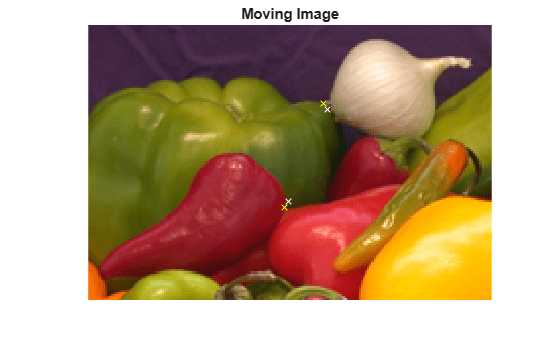# cpcorr

Tune control point locations using cross-correlation

## Syntax

``movingPointsAdjusted = cpcorr(movingPoints,fixedPoints,moving,fixed)``

## Description

example

````movingPointsAdjusted = cpcorr(movingPoints,fixedPoints,moving,fixed)` adjusts the position of moving control points, `movingPoints`, with respect to fixed control points, `fixedPoints`, using normalized cross-correlation between the moving image `moving` and the fixed image `fixed`. The `cpcorr` function returns the adjusted moving control points in `movingPointsAdjusted`.```

## Examples

collapse all

Read two images into the workspace.

```moving = imread('onion.png'); fixed = imread('peppers.png');```

Define sets of control points for both images.

```movingPoints = [118 42;99 87]; fixedPoints = [190 114;171 165];```

Display the images, and display the control points in white. The position of the moving points is slightly offset from the position of the fixed points.

```imshow(fixed) hold on plot(fixedPoints(:,1),fixedPoints(:,2),'xw') title('Fixed Image')``````figure imshow(moving) hold on plot(movingPoints(:,1),movingPoints(:,2),'xw') title('Moving Image')```Adjust the moving control points using cross correlation.

```movingPointsAdjusted = cpcorr(movingPoints,fixedPoints, ... moving(:,:,1),fixed(:,:,1))```
```movingPointsAdjusted = 2×2 115.9000 39.1000 97.0000 89.9000 ```

Display the adjusted moving points in yellow. Compared to the original moving points (in white), the adjusted points more closely match the positions of the fixed points.

`plot(movingPointsAdjusted(:,1),movingPointsAdjusted(:,2),'xy')`## Input Arguments

collapse all

Coordinates of control points in the image to be transformed, specified as an m-by-2 matrix. The two columns represent the x- and y-coordinates of the control points, respectively, in the intrinsic coordinate system of the image.

Example: `[127 93; 74 59]`

Data Types: `double`

Coordinates of control points in the reference image, specified as an p-by-2 matrix. The two columns represent the x- and y-coordinates of the control points, respectively, in the intrinsic coordinate system of the image.

Example: `[323 195; 269 161]`

Data Types: `double`

Image to be registered, specified as a numeric array.

Reference image in the target orientation, specified as a numeric array.

## Output Arguments

collapse all

Adjusted coordinates of control points in the image to be transformed, returned as a numeric matrix of the same size as `movingPoints`.

Data Types: `double`

## Tips

• The `moving` and `fixed` images must have the same scale for `cpcorr` to be effective.

• If `cpcorr` cannot correlate a pair of control points, `movingPointsAdjusted` contains the same coordinates as `movingPoints` for that pair.

• `cpcorr` cannot adjust a point if any of these conditions occur:

• points are too near the edge of either image

• regions of images around points contain `Inf` or `NaN`

• region around a point in moving image has zero standard deviation

• regions of images around points are poorly correlated

## Algorithms

`cpcorr` only moves the position of a control point by up to four pixels. Adjusted coordinates are accurate up to one-tenth of a pixel. `cpcorr` is designed to get subpixel accuracy from the image content and coarse control point selection.

## Version History

Introduced before R2006a

expand all